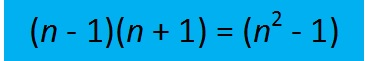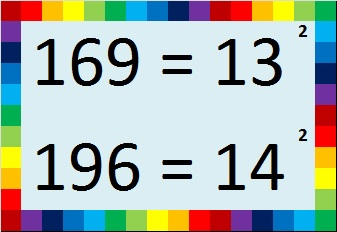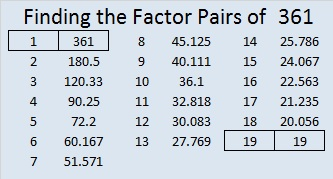# 361 Do You See a Pattern?

When 2^361 is divided by 361, the remainder is 116, not 2. That means that 361 is definitely a composite number. Its factors are listed at the end of this post.

361 isn’t used as often, but it is just as special as some of the numbers in the table below:The pattern can also be seen along the diagonals in this ordinary multiplication table:This pattern could be very helpful to students who are learning to multiply. I have seen plenty of students who knew 7 x 7 = 49, but couldn’t remember what 6 x 8 is.

Years after I learned the multiplication facts, I learned how to multiply binomials in an algebra class. I learned about the difference of two squares. In the example below one of the squares is n² and the other square is 1² which is equal to 1. I learned that the equationis true for ALL numbers, but nobody pointed out any practical examples to make it more meaningful. The table at the top of the page contains twelve practical examples. Let’s see how you do applying it to products of a few larger numbers.

Sometimes we find easy ways to remember certain products likeWe can use those products to help us remember other products easily by applying the difference of two squares. Try these: (Yes, you can easily do them without a calculator!)

• 13 x 13 = 169. How much is 12 x 14?
• 14 x 14 = 196. How much is 13 x 15?
• 20 x 20 = 400. How much is 19 x 21?
• If you know that the first ten powers of 2 are 2, 4, 8, 16, 32, 64, 128, 256, 512, 1024, then it’s easy to remember that 16 x 16 = 256. How much is 15 x 17?
• What if we go in the opposite direction…It isn’t too hard to multiply 18 x 20 in your head to get 360. How much is 19 x 19?
• 22 x 20 = 440 was also easy to find. How much is 21 x 21?
• 30 x 30 = 900. How much is 29 x 31?
• 100 x 100 = 10,000. How much is 99 x 101?Did you figure out what 361 has to do with this pattern? It is a perfect square just like 1, 4, 9, 16, and 25. Here is its factoring information:

• 361 is a composite number.
• Prime factorization: 361 = 19^2
• The exponent in the prime factorization is 2. Adding one we get (2 + 1) = 3. Therefore 361 has exactly 3 factors.
• Factors of 361: 1, 19, 361
• Factor pairs: 361 = 1 x 361 or 19 x 19
• 361 is a perfect square. √361 = 19## One thought on “361 Do You See a Pattern?”

1.Steve Morris

Fascinating! I have never heard of this before.

This site uses Akismet to reduce spam. Learn how your comment data is processed.